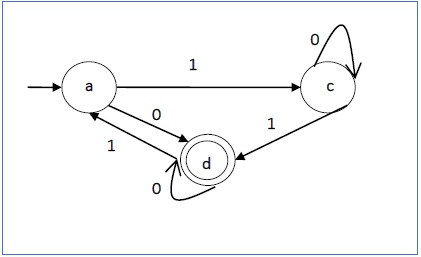# Non-deterministic Finite Automaton

#### Finite Automata

98 Lectures 10 hours

#### Theory of Computation - Finite Automata | Automata Theory in Hindi

15 Lectures 2 hours

#### State Machines and Automata: Building a RegExp Machine

16 Lectures 1.5 hours

In NDFA, for a particular input symbol, the machine can move to any combination of the states in the machine. In other words, the exact state to which the machine moves cannot be determined. Hence, it is called Non-deterministic Automaton. As it has finite number of states, the machine is called Non-deterministic Finite Machine or Non-deterministic Finite Automaton.

### Formal Definition of an NDFA

An NDFA can be represented by a 5-tuple (Q, ∑, δ, q0, F) where −

• Q is a finite set of states.

• is a finite set of symbols called the alphabets.

• δ is the transition function where δ: Q × ∑ → 2Q

(Here the power set of Q (2Q) has been taken because in case of NDFA, from a state, transition can occur to any combination of Q states)

• q0 is the initial state from where any input is processed (q0 ∈ Q).

• F is a set of final state/states of Q (F ⊆ Q).

### Graphical Representation of an NDFA: (same as DFA)

An NDFA is represented by digraphs called state diagram.

• The vertices represent the states.
• The arcs labeled with an input alphabet show the transitions.
• The initial state is denoted by an empty single incoming arc.
• The final state is indicated by double circles.

Example

Let a non-deterministic finite automaton be →

• Q = {a, b, c}
• ∑ = {0, 1}
• q0 = {a}
• F = {c}

The transition function δ as shown below −

Present State Next State for Input 0 Next State for Input 1
a a, b b
b c a, c
c b, c c

Its graphical representation would be as follows −## DFA vs NDFA

The following table lists the differences between DFA and NDFA.

DFA NDFA
The transition from a state is to a single particular next state for each input symbol. Hence it is called deterministic. The transition from a state can be to multiple next states for each input symbol. Hence it is called non-deterministic.
Empty string transitions are not seen in DFA. NDFA permits empty string transitions.
Backtracking is allowed in DFA In NDFA, backtracking is not always possible.
Requires more space. Requires less space.
A string is accepted by a DFA, if it transits to a final state. A string is accepted by a NDFA, if at least one of all possible transitions ends in a final state.

## Acceptors, Classifiers, and Transducers

### Acceptor (Recognizer)

An automaton that computes a Boolean function is called an acceptor. All the states of an acceptor is either accepting or rejecting the inputs given to it.

### Classifier

A classifier has more than two final states and it gives a single output when it terminates.

### Transducer

An automaton that produces outputs based on current input and/or previous state is called a transducer. Transducers can be of two types −

• Mealy Machine − The output depends both on the current state and the current input.

• Moore Machine − The output depends only on the current state.

## Acceptability by DFA and NDFA

A string is accepted by a DFA/NDFA iff the DFA/NDFA starting at the initial state ends in an accepting state (any of the final states) after reading the string wholly.

A string S is accepted by a DFA/NDFA (Q, ∑, δ, q0, F), iff

δ*(q0, S) ∈ F

The language L accepted by DFA/NDFA is

{S | S ∈ ∑* and δ*(q0, S) ∈ F}

A string S′ is not accepted by a DFA/NDFA (Q, ∑, δ, q0, F), iff

δ*(q0, S′) ∉ F

The language L′ not accepted by DFA/NDFA (Complement of accepted language L) is

{S | S ∈ ∑* and δ*(q0, S) ∉ F}

Example

Let us consider the DFA shown in Figure 1.3. From the DFA, the acceptable strings can be derived.Strings accepted by the above DFA: {0, 00, 11, 010, 101, ...........}

Strings not accepted by the above DFA: {1, 011, 111, ........}